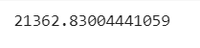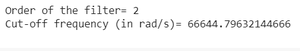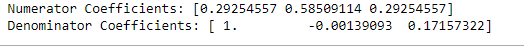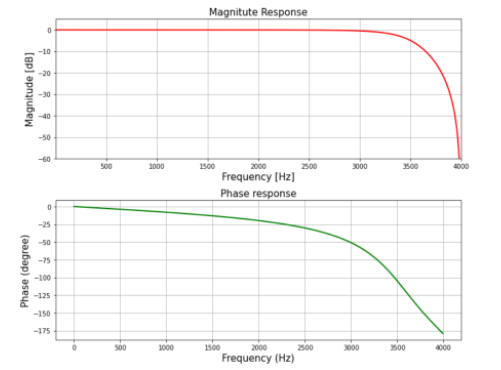Design IIR Lowpass Butterworth Filter using Bilinear Transformation Method in Scipy- Python

• Last Updated : 16 Dec, 2021

IIR stands for Infinite Impulse Response, It is one of the striking features of many linear-time invariant systems that are distinguished by having an impulse response h(t)/h(n) which does not become zero after some point but instead continues infinitely.

What is IIR Lowpass Butterworth ?

It basically behaves just like an ordinary digital Lowpass Butterworth Filter with an infinite impulse response.

The specifications are as follows:

• Sampling rate of 8 kHz
• Order of Filter 2
• Cutoff-frequency 3400Hz

We will plot the magnitude & phase response of the filter.

Step-by-step Approach:

Step 1: Importing all the necessary libraries.

Python3

 # import required libraryimport numpy as npimport scipy.signal as signalimport matplotlib.pyplot as plt

Step 2: Define variables with the given specifications of the filter.

Python3

 # Given specificationN = 2  # Order of the filterFs = 8000  # Sampling frequency in Hzfc = 3400  # Cut-off frequency in Hz # Compute Design Sampling parameterTd = 1/Fs

Step 3: Computing the cut-off frequency

Python3

 # Compute cut-off frequency in radian/secwd = 2*np.pi*fcprint(wd)  # Cut-off frequency in radian/sec

Output:Step 4: Pre-wrapping the analog frequency

Python3

 # Prewarp the analog frequency wc = (2/Td)*np.tan(wd*Td/2)print('Order of the filter=', N)  # Order # Prewarped analog cut-off frequencyprint('Cut-off frequency (in rad/s)=', wc)

Output:Step 5: Designing the filter using signal.butter() function and then performing bilinear transformation using signal.bilinear() function

Python3

 # Design analog Butterworth filter using signal.butter function b, a = signal.butter(N, wc, 'low', analog='True')# Perform bilinear Transformation z, p = signal.bilinear(b, a, fs=Fs) # Print numerator and denomerator coefficients of the filterprint('Numerator Coefficients:', z)print('Denominator Coefficients:', p)

Output:Step 6: Computing the frequency response of the filter using signal.freqz() function and plotting the magnitude and phase response

Python3

 # Compute frequency response of the filter using signal.freqz functionwz, hz = signal.freqz(z, p, 512) # Plot filter magnitude and phase responses using subplot.# Convert digital frequency wz into analog frequency in Hzfig = plt.figure(figsize=(12, 10)) # Calculate Magnitude from hz in dBMag = 20*np.log10(abs(hz)) # Calculate frequency in Hz from wzFreq = wz*Fs/(2*np.pi) # Plot Magnitude responsesub1 = plt.subplot(2, 1, 1)sub1.plot(Freq, Mag, 'r', linewidth=2)sub1.axis([1, Fs/2, -60, 5])sub1.set_title('Magnitude Response', fontsize=15)sub1.set_xlabel('Frequency [Hz]', fontsize=15)sub1.set_ylabel('Magnitude [dB]', fontsize=15)sub1.grid()  # Plot phase anglesub2 = plt.subplot(2, 1, 2) # Calculate phase angle in degree from hzPhase = np.unwrap(np.angle(hz))*180/np.pisub2.plot(Freq, Phase, 'g', linewidth=2)sub2.set_ylabel('Phase (degree)', fontsize=15)sub2.set_xlabel(r'Frequency (Hz)', fontsize=15)sub2.set_title(r'Phase response', fontsize=15)sub2.grid() plt.subplots_adjust(hspace=0.5)fig.tight_layout()plt.show()

Output:Below is the implementation:

Python3

 # import required libraryimport numpy as npimport scipy.signal as signalimport matplotlib.pyplot as plt # Given specificationN = 2  # Order of the filterFs = 8000  # Sampling frequency in Hzfc = 3400  # Cut-off frequency in Hz # Compute Design Sampling parameterTd = 1/Fs # Compute cut-off frequency in radian/secwd = 2*np.pi*fcprint(wd)  # Cut-off frequency in radian/sec # Prewarp the analog frequencywc = (2/Td)*np.tan(wd*Td/2)print('Order of the filter=', N)  # Order # Prewarped analog cut-off frequencyprint('Cut-off frequency (in rad/s)=', wc) # Design analog Butterworth filter using signal.butter functionb, a = signal.butter(N, wc, 'low', analog='True') # Perform bilinear Transformationz, p = signal.bilinear(b, a, fs=Fs) # Print numerator and denomerator coefficients of the filterprint('Numerator Coefficients:', z)print('Denominator Coefficients:', p) # Compute frequency response of the filter using signal.freqz functionwz, hz = signal.freqz(z, p, 512) # Plot filter magnitude and phase responses using subplot.#Convert digital frequency wz into analog frequency in Hzfig = plt.figure(figsize=(10, 8)) # Calculate Magnitude from hz in dBMag = 20*np.log10(abs(hz)) # Calculate frequency in Hz from wzFreq = wz*Fs/(2*np.pi) # Plot Magnitude responsesub1 = plt.subplot(2, 1, 1)sub1.plot(Freq, Mag, 'r', linewidth=2)sub1.axis([1, Fs/2, -60, 5])sub1.set_title('Magnitude Response', fontsize=15)sub1.set_xlabel('Frequency [Hz]', fontsize=15)sub1.set_ylabel('Magnitude [dB]', fontsize=15)sub1.grid() # Plot phase anglesub2 = plt.subplot(2, 1, 2) # Calculate phase angle in degree from hzPhase = np.unwrap(np.angle(hz))*180/np.pisub2.plot(Freq, Phase, 'g', linewidth=2)sub2.set_ylabel('Phase (degree)', fontsize=15)sub2.set_xlabel(r'Frequency (Hz)', fontsize=15)sub2.set_title(r'Phase response', fontsize=15)sub2.grid() plt.subplots_adjust(hspace=0.5)fig.tight_layout()plt.show()

Output:Attention geek! Strengthen your foundations with the Python Programming Foundation Course and learn the basics.

To begin with, your interview preparations Enhance your Data Structures concepts with the Python DS Course. And to begin with your Machine Learning Journey, join the Machine Learning - Basic Level Course

My Personal Notes arrow_drop_up